Maths
Study Material

# Differentiation Formulas | Differential Calculus | Full List

Here, we have provided various forms of differentiation formulas ranging from Power Functions, Trigonometric Functions, Exponential Functions etc.

4 minutes longHesap Oluştur

Got stuck on homework? Get your step-by-step solutions from real tutors in minutes! 24/7. Unlimited.

A full list of basic differentiation formulas have been provided below for the ease of students, so that they can find all the relevant differentiation formula(s) at one place.

It’s important to memorize the various formulas as it is the basis of the chapter “Differentiation”.

Here, we have provided various forms of differentiation formula(s) ranging from Power Functions, Trigonometric Functions, Exponential Functions etc.

## Differentiation of a Power Function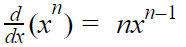Where n is a fixed number, integer or rational.

## Differentiation of a Constant Value

The differentiation of a constant value is always zero.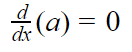## Differentiation of a Function multiplied with a constant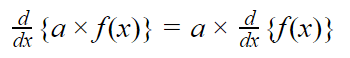## Differentiation of the Sum/Difference of Functions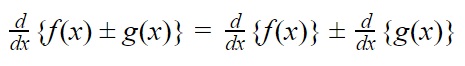## Differentiation of the Product of Functions

If f(x) and g(x) are two differentiable functions then f(x).g(x) is also differentiable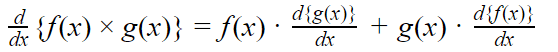## Differentiation of the Quotient of Two Functions

If f(x) and g(x) are two differentiable functions and g(x)≠0 then f(x)/g(x) is also differentiable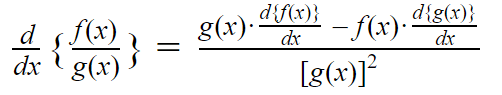## Differentiation Formulas for Trigonometric Functions

Trigonometric functions can be defined as the functions of an angle of a triangle. The basic trigonometric functions are sine, cosine, tangent, cotangent, secant and cosecant. To learn more about Trigonometry and its formulas check out Trigonometry Formula(s).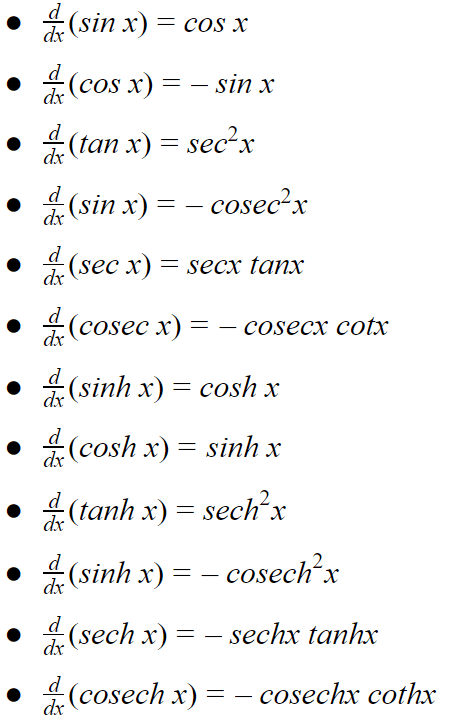## Differentiation Formulas for Inverse Trigonometric Functions

Inverse trigonometric functions are basically the inverse of the basic trigonometric functions i.e. sine, cosine, tangent, cotangent, secant, and cosecant functions. Differentiation of these inverse trigonometric functions are given below: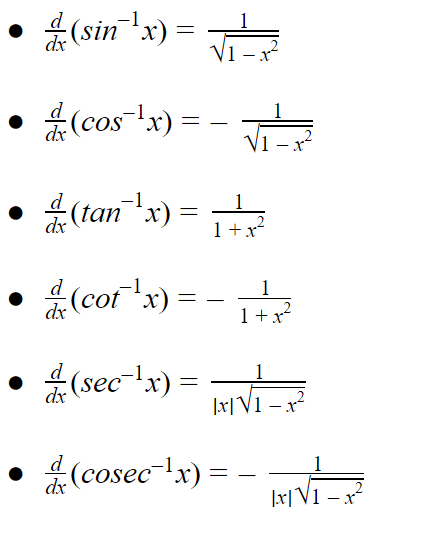## Exponential / Logarithmic Differentiation Formulas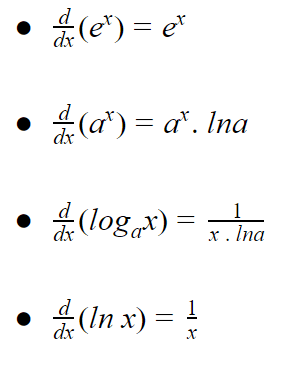## Differentiation Formulas of Function of a Function

### CHAIN RULE

If y = f(t) and t = g(x) then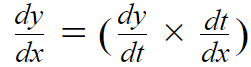This rule can be extended further.

If  y = f(t) and t = g(u) and u = h(x) then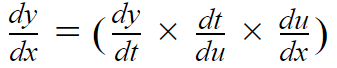## Picked For You

Furthermore, these are some topics which might interest you: